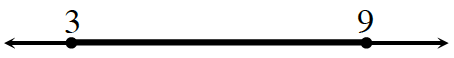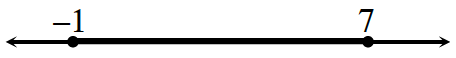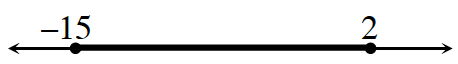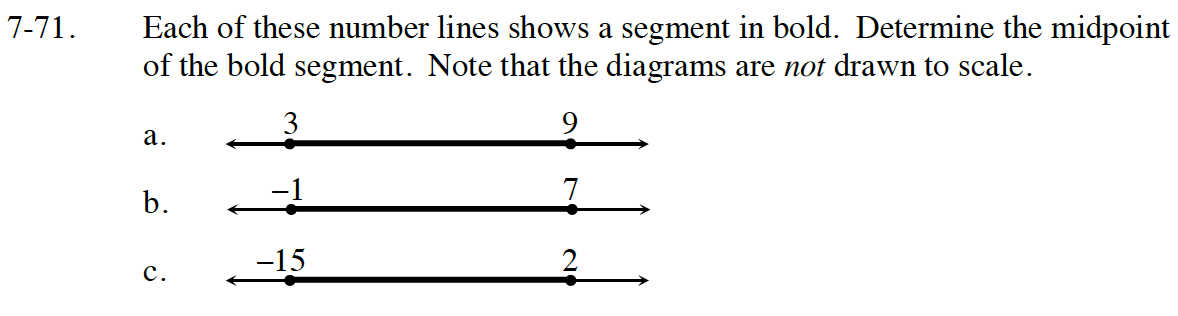Home > CC4 > Chapter 7 > Lesson 7.1.6 > Problem7-71

7-71.
1. Each of these number lines shows a segment in bold. Determine the midpoint of the bold segment. Note that the diagrams are not drawn to scale. Homework Help ✎

1.2.3.The midpoint is the mean, or average. What is the mean of each of these pairs of numbers?

$\frac{\left(3+9\right)}{2}=6$